# Rectangles

How many different rectangles with sides integers (in mm) have a circumference exactly 1000 cm? (a rectangle with sides of 50cm and 450cm is considered to be the same as a rectangle with sides of 450cm and 50cm)

n =  2500

### Step-by-step explanation: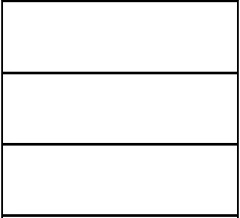Did you find an error or inaccuracy? Feel free to write us. Thank you!Tips to related online calculators
Do you want to convert length units?

#### You need to know the following knowledge to solve this word math problem:

We encourage you to watch this tutorial video on this math problem:

## Related math problems and questions:

• TailorTailor has an estimated 11 meters of 80 cm wide fabric. He will shape wholly 7 rectangles measuring 80 x 150 cm and nothing fabric does not remain. How many fabric he have?
• Two rectanglesI cut out two rectangles with 54 cm², 90 cm². Their sides are expressed in whole centimeters. If I put these rectangles together I get a rectangle with an area of 144 cm2. What dimensions can this large rectangle have? Write all options. Explain your calc
• Do you solve this?Determine area S of rectangle and length of its sides if its perimeter is 102 cm.
• Square into three rectangles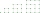Divide the square with a side length of 12 cm into three rectangles with have the same circumference so that these circumferences are as small as possible.
• Rhombus sidesfind the sides of a rhombus, it area is 550 cm square and altitude is 55cm
• TableclothsThe restaurant has sixty-two square tablecloths with a side length of 150 cm and 36 rectangular tablecloths with dimensions of 140 cm and 160 cm. A) How many meters of hemming ribbon will be needed if we add 50 cm to each tablecloth? B) The ribbon sale in
• Tablecloth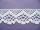The table top is 90 cm long and 50 cm wide. How many cm lace edging is necessary for the tablecloth that hangs on each side by 10 cm?
• RectanglesVladimir likes to draw rectangles. Yesterday he created all rectangles that had sides in centimeters and a circumference of 18 cm. How many rectangles of different dimensions have been drawn?
• The bookThe book has 280 pages. Each side is a rectangle with sides 15 cm and 22 cm. a) how many leaves has the book? b) at least how many square meters of paper should be used to production this book?
• Table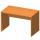Find the circumference of the table, where the long side is 1.28 meters and the short side is 86 cm.
• Rope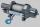How many meters of rope 10 mm thick will fit on the bobbin diameter of 200 mm and length 350 mm (central mandrel have a diameter 50 mm)?
• Steps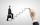An adult step has a length 76 cm. How many steps will he go distance 50 meters? How many meters does he go when he makes 700 steps?
• Fence of the garden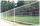The garden has dimensions of 5m and 400cm. How many meters meshes are needed for fencing the plot?
• ThalesThales is 1 m from the hole. The eyes are 150 cm above the ground and look into the hole with a diameter of 120 cm as shown. Calculate the depth of the hole.
• RectangleThe length of one side of the rectangle is three times the length of the second side. What are the dimensions of the rectangle if its circumference 96 cm?
• Painter 3Dad want to paint wall high 250 cm wide and 230 cm with wallpaper. How many meters must buy wallpaper if wallpaper width is 60 cm?
• Two sides paintThe door has the shape of a rectangle with dimensions of 260cm and 170cm. How many cans of paint will be needed to paint this door if one can of paint cover 2m2 of the area? We paint the doors on both sides.